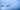# Enabling Tensorboard to see what's happening during training of an LSTM on Keras using a Timeseries Generator

March 30, 2018

Learning neural networks is still very messy, so without tools such as tensorboard, it would be really hard to really comprehend what is happening. Unfortunately, it's not super easy to get tensorboard working out-of-the-box with Keras when training certain structures.

When running an LSTM learning algorithm on large datasets, it is sometimes needed to generate the sequences on the fly and feed the data that is used to learn the algorithms in several steps. If the size of the data is large but the series for each logical unit is small however, it is often not possible to run several epochs on the whole set but rather run single epochs on each smaller unit.

In my specific case I was analyzing some 3GB of sequential forecast data and its corresponding realized data values. To learn from historical data, it is necessary to let the LSTM see anything from up to 7 days before the current reading. That equates to each sequence being equal to about \$24 * 7\$ the size of the original data. That is clearly too much to generate ahead of time and then just feed to the CPU from memory. So a different approach is needed. Because the whole dataset cannot be fed to the LSTM directly however, I am using the `model.fit_generator` API of Keras. This allows me to pass a `Sequence` object that generates Sequences from an underlying data array on the fly. This API doesn't support the `validation_split` flag, which requires me to pass a separate generator to the API for its validation steps. However, this is not supported by Tensorflow (no generators allowed), which requires me to create a separate Tuple of (X,Y) values that is in-memory and that can be used for validation. Since this doesn't need to be so large, it fits into memory.

My overall learning structure sort of looks as such

``````def run_full_learning():
# iterate over games (holding customer lists)
for g_number, game in enumerate(preprocessing.GamesIterator()):
# iterating over customers in game
# sequences is [generator, validation_set]
for sequences in preprocessing.CustomerIterator(game, game):
model.fit_with_generator(mdl, sequences, sequences)
mdl.save_weights("data/consume/game{}.HDF5".format(g_number))``````

I train my model on every customer sequence which is some 2000 timesteps long and which has 17 features per timestep. Every game holds about 200 customers and I have about 100 games. After training on every customer, tensorboard gets to validate and calculate the loss after each epoch is done (and each epoch is one customer set being completed). The exact train call of Keras is this:

``````model.fit_generator(train_generator,
steps_per_epoch=None,  #a generator size (aka one customer) is an epoch
epochs=1,
verbose=2,  #progress bar, 2 = line per epoch
callbacks=callbacks,
validation_data=validation_set,
#validation_steps=None,
class_weight=None,
max_queue_size=10,
workers=8,
use_multiprocessing=True,
shuffle=True,
initial_epoch=0)``````

To generate the validation set, I first used the `util.TimeseriesGenerator` to generate the sequences. But since I
don't actually want to feed a generator to tensorflow, I instead converted it back into an array that I can give to Keras. This way, Tensorboard is happy about the fact that it is not receiving a generator and I am happy because I get visual feedback.

``````	def generate_batches_for_customer(data, targets):
"""[keras docs](https://keras.io/preprocessing/sequence/#timeseriesgenerator)"""
return TimeseriesGenerator(data,
targets,
length=SEQUENCE_LENGTH,
sampling_rate=SAMPLING_RATE,
batch_size=BATCH_SIZE)
#...

make_sequences(self, cutofa, data, targets):
"""given a cutoff and two data arrays, generate Sequences from it (one before cutoff one after"""
training_sequence   = generate_batches_for_customer(np.array(data[:-cutoff]), np.array(targets[:-cutoff]))
validation_sequence = generate_batches_for_customer(np.array(data[-cutoff:]), np.array(targets[-cutoff:]))
return training_sequence, validation_sequence

def get_next_pair(self):
"""pops the first of both arrays out and returns it"""
data    = self.data_customers.pop(0)
targets = self.targets_customers.pop(0)
return data, targets

def convert_sequence_to_set(self, sequence: Sequence):
#this is a set of tuples. We need a tuple of sets...
x = []
y = []
for i in range(sequence.length):
batch = sequence[i]
x.extend(batch)
y.extend(batch)
return np.array(x), np.array(y)``````

So to sum it up, it is possible to get Tensorboard going with util.Sequence from a TimeseriesGenerators, but it's a little messy as we first make a Sequence object and then drain it of all sequences to pass directly to the train method. I guess a future API update will allow Sequence objects also when using a Tensorboard callback but for now this will do.